Starts With A Bang

# This One Equation, 10² + 11² + 12² = 13² + 14², Takes Pythagoras To A Whole New Level

#### Unbelievably enough, it all comes back to Pythagoras.

One of the first theorems anyone learns in mathematics is the Pythagorean Theorem: if you have a right triangle, then the square of the longest side (the hypotenuse) will always equal the sums of the squares of the other two sides. The first integer combination that this works for is a triangle with sides 3, 4, and 5: ³² + ⁴² = ⁵². There are other combinations of numbers that this works for, too, including:

• 5, 12, and 13,
• 6, 8, and 10,
• 7, 24 and 25,

and infinitely more. But 3, 4, and 5 are special: they’re the only consecutive whole numbers that obey the Pythagorean Theorem. In fact, they’re the only consecutive whole numbers that allow you to solve the equation a² + b² = c² at all. But if you allowed yourself the freedom to include more numbers, you could imagine that there might be consecutive whole numbers that worked for a more complex equation, like a² + b² + c² = d² + e². Remarkably, there’s one and only one solution: 10² + 11² + 12² = 13² + 14². Here’s why.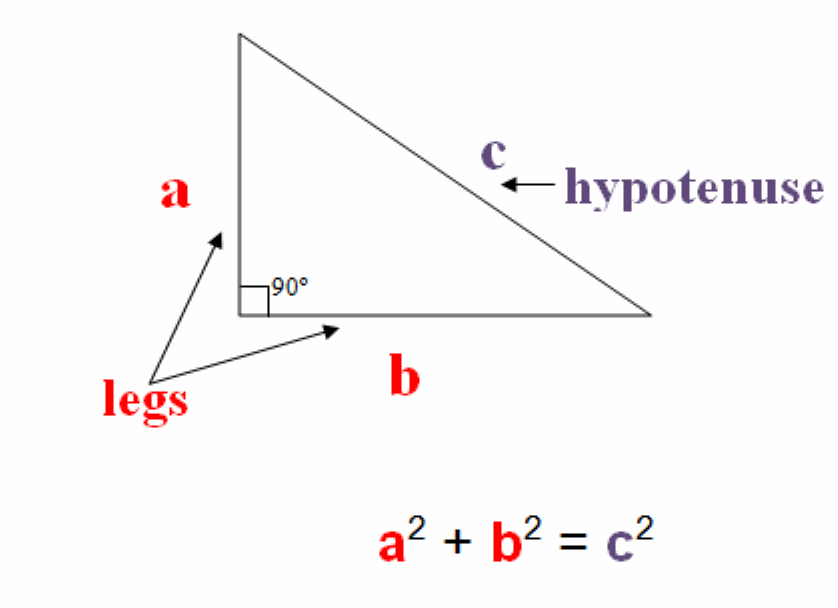If you take the sum of the squares of any two legs of any right triangle, it will always equal the square of the hypotenuse. But there is much more to this relation than a simple equation. (HISTORYOFPYTHAGOREANTHEOREM.WEEBLY.COM)

One of the most profound way to look at the Pythagorean Theorem is to think about a square that’s a certain length on all sides: let’s call that length b. The area of that square is b², because the length and the width of that square get multiplied by each other. If we want to make it so that a² + b² = c², and we want a, b, and c to all be consecutive numbers, then that puts tremendous restrictions on a and c.

It means that c has to equal (b + 1) and that a has to equal (b — 1), and that’s an equation we can solve with just a little algebra.

(b — 1)² + (b)² = (b + 1)²,

b² — 2b + 1 + b² = b² + 2b + 1

b² — 4b = 0.

And therefore, b has to equal 0 (which isn’t interesting) or 4, where 4 gives us back our old Pythagorean solution of 3² + 4² = 5².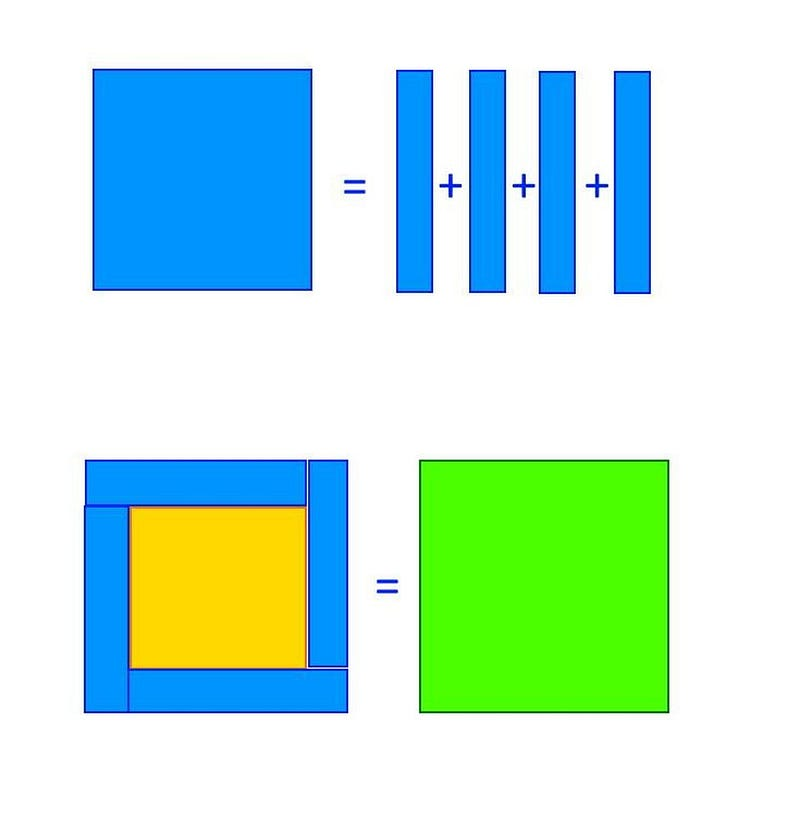At the top, a square of side “b” (blue) can be broken up into four segments. If you stack them properly along the sides of a square of side length “b-1” (yellow), you can wind up with a square of side length “b+1” (green), another way to illustrate the Pythagorean theorem. (E. SIEGEL)

But you could also solve this graphically. If you start with a square that’s b on all sides, then you can break it up into lines that are each 1 unit thick. Because a square has 4 sides, the only way you’ll be able to add those lines to a smaller square [that’s (b — 1) on all sides] and wind up with a larger square [that’s (b + 1) on all sides] is if you have 4 segments: one to add onto each side.Travel the Universe with astrophysicist Ethan Siegel. Subscribers will get the newsletter every Saturday. All aboard!

The above image clearly shows how to do this:

• you break up the middle square into b chunks of 1 unit each,
• you stack the chunks around the smaller square [of size a, which is (b — 1)],
• and wind up with a larger square [of size c, which is (c + 1)].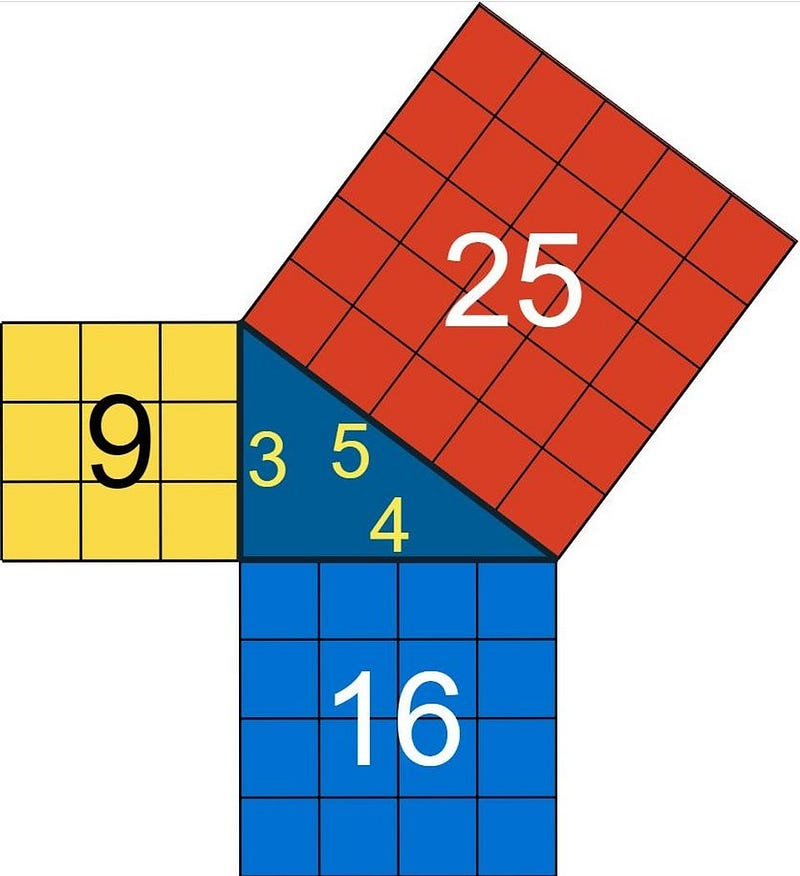The 3, 4, 5 right triangle, the first set of integers to satisfy the Pythagorean theorem, is also the only set of consecutive whole numbers that satisfies that equation. (MATHSISFUN.COM)

This is the only solution of consecutive whole numbers that works for the equation a² + b² = c². If you made your mid-sized square any larger or smaller, you would have the wrong number of lines to place around a smaller square to grow it into a larger square; it simply can’t be done. For a² + b² = c², the consecutive whole numbers of 3, 4, and 5 are the only ones that work.

But why restrict yourself to only three numbers? It’s possible that you might find consecutive whole numbers that satisfied this type of relationship for any odd number of consecutive whole numbers, such as:

• a² + b² = c²,
• a² + b² + c² = d² + e²,
• a² + b² + c² + d² = e² + + ,

and so on.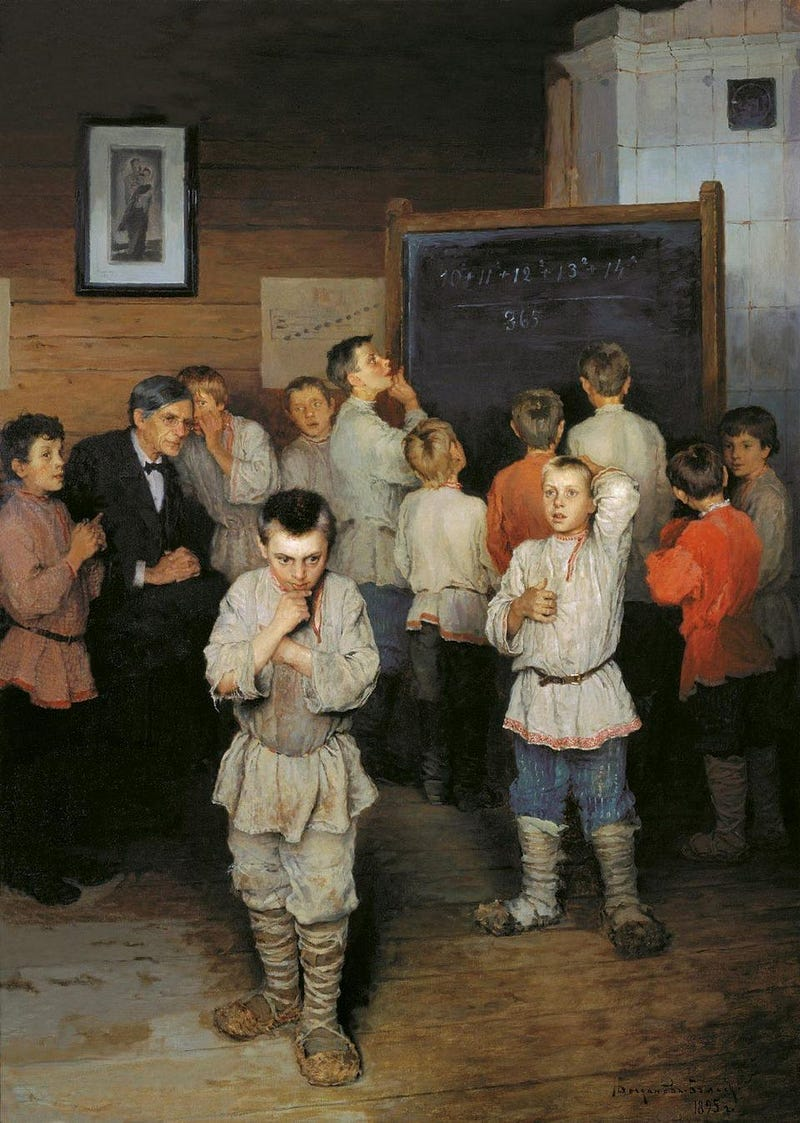The equation 1⁰² + 1¹² + 1²² = 1³² + 1⁴², whose answer is that both sides equal 365, was immortalized in a different form in this 1895 painting: “Mental Arithmetic. In the Public School of S. Rachinsky.” (NIKOLAY BOGDANOV-BELSKY)

In fact, if you look at the second possibility, where a² + b² + c² = d² + e², you’ll find that there’s one and only one combination of numbers that works: 10² + 11² + 12² = 13² + 14². This works out to 100 + 121 + 144 on the left side, which adds up to 365, and 169 + 196 on the right side, which also adds up to 365.

If you were intent on solving this type of equation with algebra, you’d still be able to do it, but it might take a little while. You’d eventually wind up with figuring out that the middle number, c, had to be 12 (or 0, which again isn’t interesting), and therefore the full equation that works is 10² + 11² + 12² = 13² + 14².

But if we went back to that same graphical approach from earlier, we could find the solution in a remarkably intuitive way.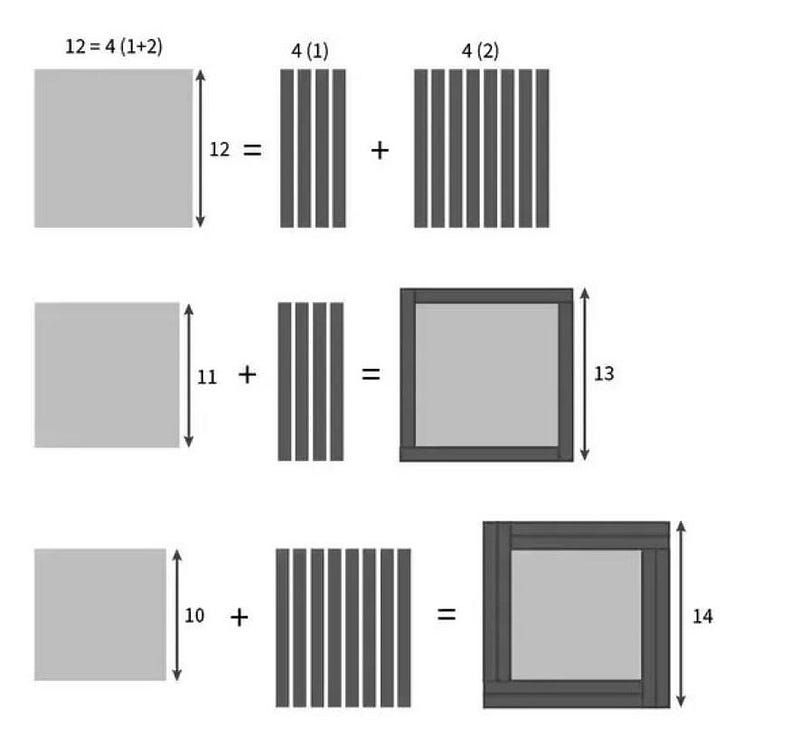Similarly, if we want to deconstruct a square and use it to turn two smaller squares into two larger squares, we need 4 units to adjust the square-size by 2 and 8 units to adjust the square-size by 4. This means that a square of size 12 can turn a square of 11 and 10 units, respectively, into squares of 13 and 14 units. (FERMAT’S LIBRARY, VIA HTTPS://TWITTER.COM/FERMATSLIBRARY/STATUS/887668606712115201)

Just like before, we’re going to take the middle “square” (where all its sides are of length c) and break it up into lines that are 1 unit thick. Unlike the first time we did this trick, however, this time we have two squares that we need to turn into larger squares using these lines:

1. turning a smaller square [where its sides are (c — 1)] into a larger square [whose sides are all (c + 1)], and
2. turning an even smaller square [whose sides are all (c — 2)] onto a still larger square [whose sides are all (c + 2)].

To accomplish this for the first square, just like last time, we need a total of four lines that are 1 unit thick to accomplish this. But to accomplish this for the second square, we need four lines that are 2 units thick.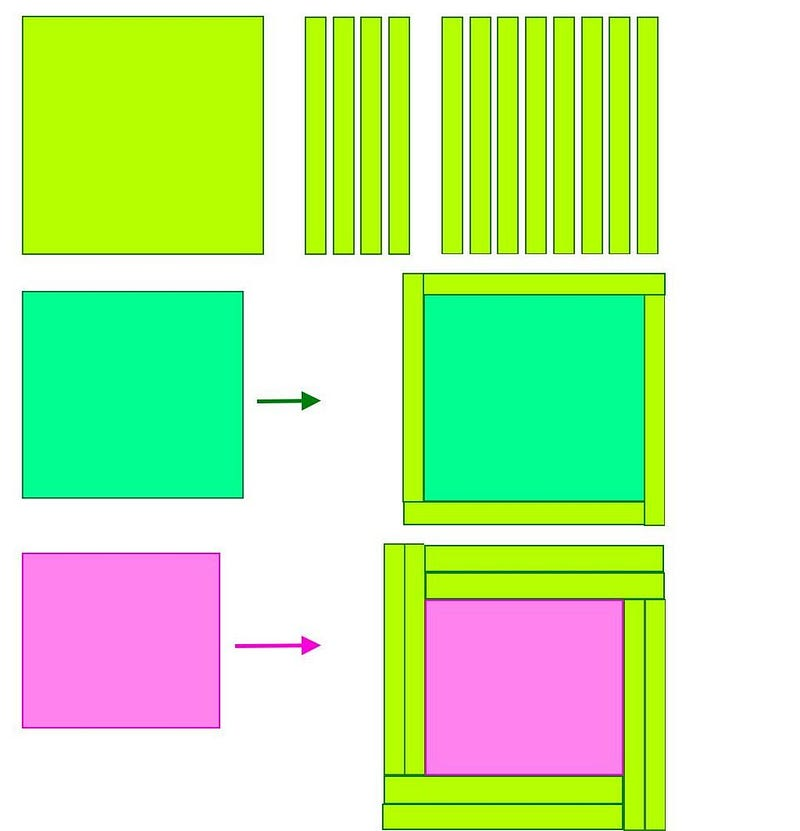If we want to use a square of size “c” to turn two smaller squares (c-1) and (c-2) into two larger squares of size (c+1) and (c+2), we need 12 units to be in that mid-sized square to make it happen. (E. SIEGEL)

All told, this only works if the thickness of that middle “square” is 12 units thick, and that’s why we get the equation 10² + 11² + 12² = 13² + 14². If you have a line that’s 12 units by 1 unit, then you can take four of them (4 × 12 = 48) and transform 11² into 13², since 121 + 48 = 169. Similarly, you could take eight such lines (8 × 12 = 96), and transform 10² into 14², since 100 + 96 = 196. This is the only solution of consecutive whole numbers to the equation a² + b² + c² = d² + e².

At this point, you might start to see a pattern emerge, which is always interesting from a mathematical perspective. We can see it much more clearly if we take the next step and ask what the solution would be for the continuation of this equation to include even more numbers.

In other words, how would we find the solution to the equation, a² + b² + c² + d² = e² + + ?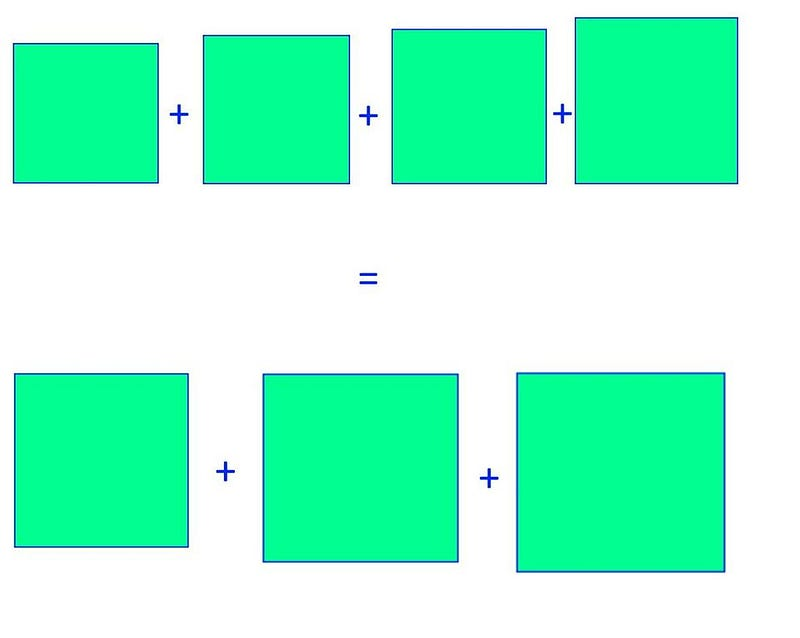Taking the sum of four consecutive perfect squares and requiring them to equal the sum of the next three perfect squares is the third possible equation we can write down representing a Pythagorean Run. (E. SIEGEL)

If we take the analogous approach, there are now three smaller squares we need to turn into larger squares:

1. a square of sides (d — 1) needs to turn into a square of sides (d + 1), requiring four units of length d,
2. a square of sides (d — 2) needs to turn into a square of sides (d + 2), requiring eight units of length d, and
3. a square of sides (d — 3) needs to turn into a square of sides (d + 3), requiring twelve units of length d.

Given, now, that we need that middle “square” to have a length of 4 + 8 + 12 = 24, which gives us something that we suspect should be our solution to this equation. If it’s right, then 21² + 22² + 23² + 24² = 25² + 26² +27². When we do the math, we see that this gives us 441 + 484 + 529 + 576 = 625 + 676 + 729, which checks out. Both sides are equal to 2030, meaning they’re equal to each other.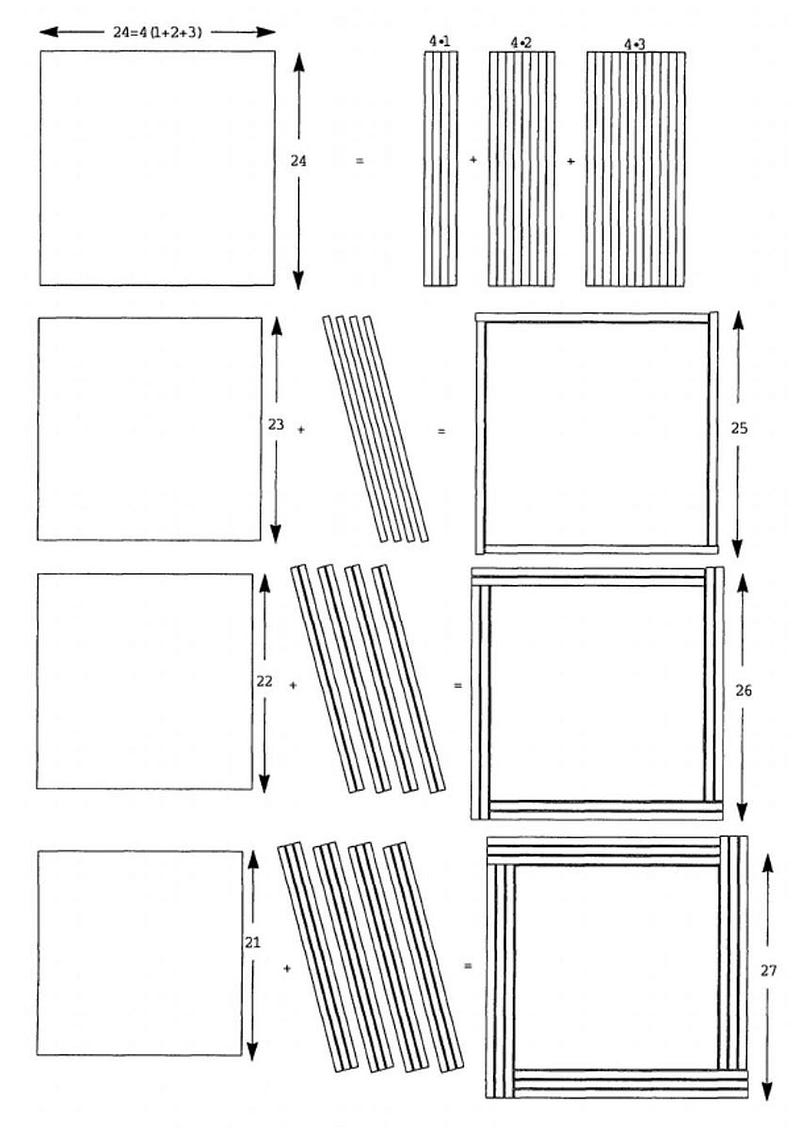This graphical illustration of the third Pythagorean Run, which is a solution to the equation a² + b² + c² + d² = e² + f² + g², illustrates why 24 is the crucial number to have for the middle square. (M. BOARDMAN, MATHEMATICS MAGAZINE (2000), V. 73, 1, P. 59)

There’s a special name for these types of sequences in mathematics that hearkens all the way back to the Pythagorean Theorem and the original solution of 3² + 4² = 5²: Pythagorean Runs. The pattern that emerged for what the middle number in the sequence is holds all the way to infinity, as it goes 4, 12, 24, 40, 60, 84, 112, etc. So if you wanted to know what the next sequences of numbers that satisfied these types of equations were, you’d wind up with:

• 36² + 37² + 38² + 39² + 40² = 41² + 42² + 43² + 44²,
• 55² + 56² + 57² + 58² + 59² + 60² = 61² + 62² + 63² + 64² + 65²,
• 78² + 79² + … + 83² + 84² = 85² + 86² + … + 89² + 90²,

and so on. What looks like a wild mathematical coincidence actually has a deep but straightforward explanation.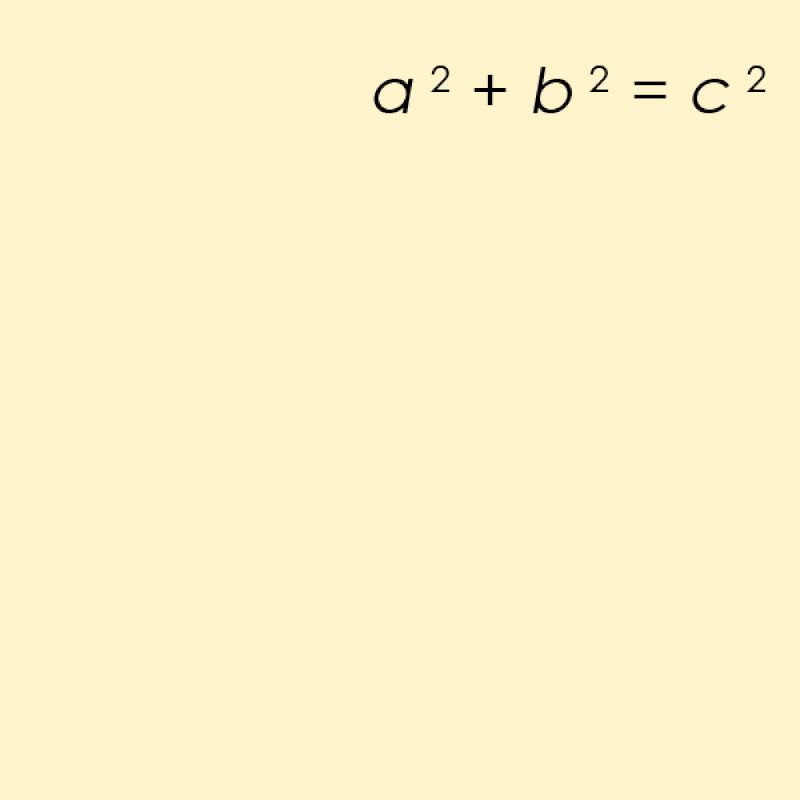There are many ways to solve and visualize a simple Pythagorean equation like a² + b² = c², but not all visualizations are equally useful when it comes to extending that equation in various mathematical ways. (AMERICANXPLORER13 AT ENGLISH WIKIPEDIA)

There are 365 days in a (non-leap) year, and 10² + 11² + 12² = 13² + 14² = 365. However, this mathematical fact doesn’t have anything to do with our calendar at all, nor with our planet’s rotation and revolution around the Sun. Instead, the number of days in a year is pure coincidence here, but the mathematical relation is a direct consequence of Pythagorean geometry, something far easier to visualize than just algebra.

Pythagoras just started with a² + b² = c², which has 3, 4, and 5 as the only set of consecutive numbers that solve it. We can extend this as long as we like, however, and for each equation with an odd number of terms we can write down, there’s only one unique solution of consecutive whole numbers. These Pythagorean Runs have a clever mathematical structure governing them, and by understanding how squares work, we can see why they couldn’t possibly behave in any other way.

Ethan Siegel is the author of Beyond the Galaxy and Treknology. You can pre-order his third book, currently in development: the Encyclopaedia Cosmologica.

Related

# Why do we so consistently underestimate progress?

Three reasons why a radically better future is more likely than we think.

# Electric planes are coming: Get ready for battery-powered flights

Short-hop regional flights could be running on batteries in a few years.

# 50 years ago, an artist convincingly exhibited a fake Iron Age civilization

The artifacts were often made from found objects – an Ivory dish-soap bottle transformed into an earthenware figure.

# One ship in two centuries: the strange tale of the SS Warrimoo

On New Year’s Eve 1899, the captain of this Pacific steamliner sailed into history. Or did he?

# Humans are not “tribal”

From politics to culture, we blame “tribalism” for humanity’s problems. This explanation is entirely wrong.

Up Next

# How to manage self-inflicted stress

Stress and anxiety therapist Dr. Amelia Aldao suggests waiting 60 seconds before reacting to a stressor, giving your rational mind time to catch up to your emotions.